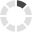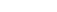### Site InformationLoading... Please wait...

# MATH 221 MATH221 Week 8 Final Exam

\$20.00

## Product Description

MATH 221 Week 8 Final Exam

1. Determine whether the following are nominal, ordinal, interval, and ratio.

2. The following numbers represent the weights in pounds of six 7-year old children in Mrs. Jones' 2nd grade class. {25, 60, 51, 47, 49, 45} Find the mean; median; mode; variance; standard deviation.

3. If the variance is 846, what is the standard deviation?

4. If we have the following data:  34, 38, 22, 21, 29, 37, 40, 41, 22, 20, 49, 47, 20, 31, 34, 66.  Draw a stem and leaf. Discuss the shape of the distribution.

5. Find the regression equation of the following data.

6. To predict the annual rice yield in pounds we use the equation yˆ 859 5.76x3.82x2  where x1 represents the number of acres planted (in thousands) and where 2x represents the number of acres harvested (in thousands) and where r2 = 0.94.

7. The Student Services office did a survey of 500 students with the following results:

8. A shipment of 40 television sets contains 3 defective units. How many ways can a vending company can buy five of these units and receive no defective units?

9. A company claims that 61% of consumers know about their product.  If the company asks 18 consumers, what is the probability that (a) exactly 10 will say yes, that (b) between 10 and 12 will say yes.

10. The mean number of cars per minute going through the Eisenhower turnpike automatic toll is about 7. Find the probability that exactly 3 will go through in a given minute.

11. On a dry surface, the braking distance (in meters) of a certain car is a normal distribution with mu = μ = 45.1 m and sigma = σ = 0.5

12. A sample of 58 days of gas prices has a mean of \$2.75 and a standard deviation of \$0.86.  Construct the 95% confidence interval.

13. Determine the minimum required sample size if you want to be 80% confident that the sample mean is within 2 units of the population mean given sigma = 9.4. Assume the population is normally distributed.

14. A social service worker wants to estimate the true proportion of pregnant teenagers who miss at least one day of school per week on average. The social worker wants to be within 5% of the true proportion when using a 90% confidence interval. A previous study estimated the population proportion at 0.21.

15. A restaurant claims that its speed of service time is less than 15 minutes. A random selection of 49 service times was collected, and their mean was calculated to be 14.5 minutes. Their standard deviation is 2.7 minutes. Is there enough evidence to support the claim at alpha = .07?  Write the hypotheses, the p-value, conclusion, and implication for the claim.

16. A doctor claims that 30% of patients only have a cold.  During one month, the doctor finds that 112 of 420 patients only has a cold.  At α=0.06, can the doctor support the claim?  Write the hypotheses, the p-value, conclusion, and implication for the claim.

## Customers also viewed

• \$15.00• \$14.99• \$14.99• \$25.00• \$14.99## Related Products

• MATH 221 MATH221 Week 5 Homework \$15.00• MATH 221 MATH/221 MATH221 Week 2 iLab DEVRY \$14.99• MATH 221 MATH/221 MATH221 Week 4 iLab DEVRY \$14.99• MATH 221 MATH/221 MATH221 Week 6 iLab DEVRY \$14.99• MATH 302 Week 8 Final Exam \$25.00Click the button below to add the MATH 221 MATH221 Week 8 Final Exam to your wish list.